# 9th Class Maths Formulas Chapter 12 Herons Formula Download in pd

## 9th Class Maths Formulas Chapter 12 Heron's Formula Download in pdf

##### • Triangle with base 'b' and altitude 'h' is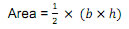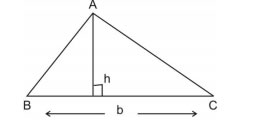##### • Triangle with sides a, b and c (i) Semi perimeter of triangle s =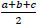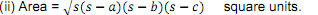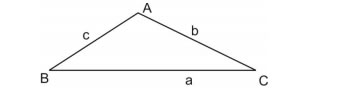##### • Equilateral triangle with side 'a'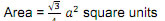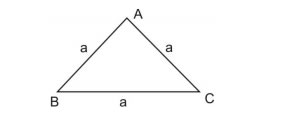##### • Trapezium with parallel sides 'a' and 'b' and the distance between two parallel sides as 'h'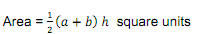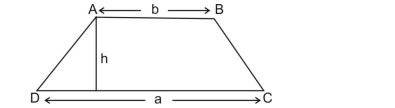Section - A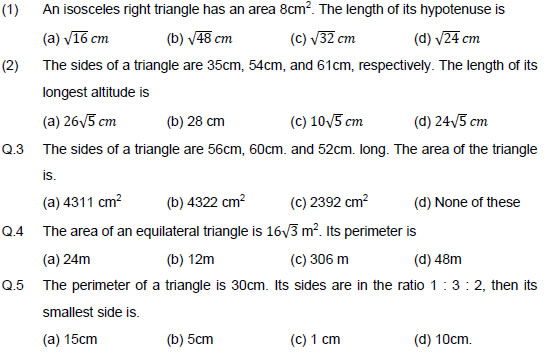Section - B

Q.6 Find the area of a triangular garden whose sides are 40m., 90m and 70m.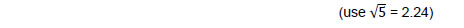Q.7 Find the cost of leveling a ground in the form of a triangle with sides 16m, 12m and 20m at Rs. 4 per sq. meter.

Q.8 Find the area of a triangle, two sides of which are 8cm and 11cm and the perimeter is 32 cm.

Q.9 The area of an isosceles triangle is 12cm2. If one of its equal side is 5cm. Find its base.

Q.10 Find the area of a right triangle whose sides containing the right angle are 5cm and 6cm.

Q.11 Find the area of the adjoin figure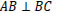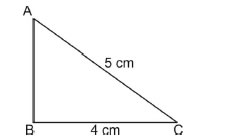Section - C

Q.12 The diagonals of a rhombus are 24cm and 10cm. Find its area and perimeter.

Q.13 Two side of a parallelogram are 10cm and 7cm. One of its diagonals is 13cm. Find the area.

Q.14 A rhombus shaped sheet with perimeter 40 cm and one diagonal 12cm, is painted on both sides at the rate of ` 5 per m2. Find the cost of painting.

Q.15 The sides of a quadrilateral ABCD are 6cm, 8cm, 12cm and 14cm (taken in order) respectively, and the angle between the first two sides is a right angle. Find its area.

Q.16 The perimeter of an isosceles triangle is 32cm. The ratio if the equal side to its base is 3 : 2. Find the area of the triangle

Q.17 The sides of a triangular field are 41m, 40m and 9m. Find the number of flower beds that can be prepared in the field, if each flower bed needs 900cm2 space.

Q.18 The perimeter of a triangular ground is 420m and its sides are in the ratio 6 : 7 : 8. Find the area of the triangular ground.

Section - D

Q.19 Calculate the area of the shaded region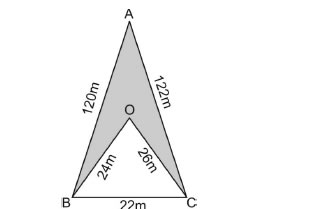Q.20 If each sides of a triangle is double, then find the ratio of area of the new triangle thus formed and the given triangle.

Q.21 A field is in the shape of a trapezium whose parallel sides are 25m and 10m. If its non-parallel sides are 14m and 13m, find its area

Q.22 An umbrella is made by stitching 10 triangular pieces of cloth of 5 different colour each piece measuring 20cm, 50cm and 50cm. How much cloth of each colour is required for one umbrella?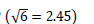Q.23 A triangle and a parallelogram have the same base and some area. If the sides of the triangle are 26cm, 28cm and 30cm and the parallelogram stands on the base 28cm, find the height of the parallelogram.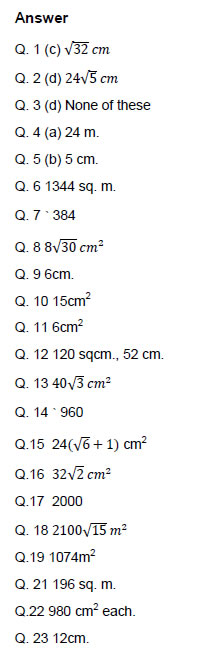### NCERT Books Free Pdf Download for Class 5, 6, 7, 8, 9, 10 , 11, 12 Hindi and English Medium

 Mathematics Biology Psychology Chemistry English Economics Sociology Hindi Business Studies Geography Science Political Science Statistics Physics Accountancy